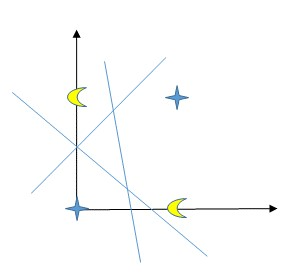@ArrowLLL 2017-07-31T16:44:18.000000Z 字数 3926 阅读 5632

# 统计学习方法 —— 感知机

机器学习

Elon Lin

## 感知机学习算法

### 原始形式

1. 选取初值 $w_0, b_0$
2. 在训练集中选取数据 $(w_i, y_i)$
3. 如果 $y_i(w \cdot x_i + b) \leq 0$
4. 转至 （2），直到训练集中没有误分类点。

### 算法收敛性

Novlkoff 定理

1. 存在满足条件 $\|\hat{w}_{opt}\| = 1$ 的超平面 $\hat{w}_{opt} \cdot \hat{x} = w_{opt} \cdot x + b_{opt} = 0$ 将训练数据集完全正确分开：且存在 $\gamma > 0$，对所有 $i = 1, 2, ..., N$

2. 令 $R = \max \limits_{1 \leq i \leq N}\|\hat{x}_i\|$，则感知机算法原始形式在训练数据集上的误分类次数k满足不等式

### 对偶形式

1. $\alpha \leftarrow 0, b \leftarrow 0$
2. 在训练集中选取数据 $x_i, y_i$
3. 如果 $y_i(\sum_{j = 1}^N\alpha_jy_jx_j \cdot x_i + b) \leq 0$
4. 转至 2 直到没有误分类数据

## 习题

1. Minsky 和 Papert 指出：感知机因为是线性模型，所以不能表示复杂的函数，如异或(XOR)。验证感知机为什么不能表示异或

已知异或表示对于同一集合的两个元素(a, b)，a和b相同则为0， 相异则为1，由此可以得到其运算关系:

• (0, 0) = (1, 1) = 0
• (0, 1) = (1, 0) = 1
可得图像 :由图像可以看出，XOR的训练集线性不可分，而感知机模型并不能学习线性不可分函数(linear insparable function)
2. 证明以下定理：样本集线性可分的充分必要条件是正实例点集所构成的凸壳与负实例点所构成的凸壳互不相交。

必要性： 假设样本集T线性可分，则存在一个超平面 $w_{opt}$ 将数据集的正实例点和负实例点完全正确地划分到 $w_{opt}$ 的两侧。显然两侧的点分别构成的凸壳不相交；
充分性： 假设存在两个凸壳A、B相交，且存在超平面 $w$ 将A和B线性分割。令A在B的凸壳内部的点为a，因为线性可分，则A中不存在两点之间的连线与超平面 $w$ 相交，而凸壳B中任意一点与A中的点的连线均与超平面 $w$ 相交，则B内部的点a也与A中任一点之间的连线不与 $w$ 相交，与A中不存在两点之间的连线与超平面小脚矛盾。故只有正负实例点所构成的两个凸壳不相交时样本集才线性可分。• 私有
• 公开
• 删除